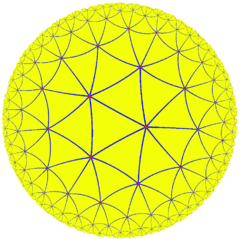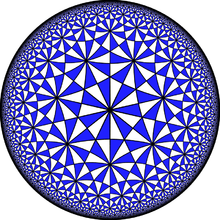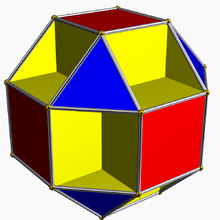# Order-7 triangular tiling

﻿
Order-7 triangular tiling
Order-7 triangular tilingPoincaré disk model of the hyperbolic plane
Type Regular hyperbolic tiling
Vertex figure 37
Schläfli symbol(s) {3,7}
Wythoff symbol(s) 7 | 3 2
Coxeter-Dynkin(s)Coxeter group [7,3]
Dual Order-3 heptagonal tiling
Properties Vertex-transitive, edge-transitive, face-transitive

In geometry, the order-7 triangular tiling is a regular tiling of the hyperbolic plane with a Schläfli symbol of {3,7}.

## Related polyhedra and tiling

This tiling is topologically related as a part of sequence of regular polyhedra with Schläfli symbol {3,p}.

The dual tiling is the order-3 heptagonal tiling.

Wythoff constructions yields further uniform tilings, yielding eight uniform tilings, including the two regular ones given here.

## Hurwitz surfacesThe symmetry group of the order-7 triangular tiling has fundamental domain the (2,3,7) Schwarz triangle, which yields this tiling.

The symmetry group of the tiling is the (2,3,7) triangle group, and a fundamental domain for this action is the (2,3,7) Schwarz triangle. This is the smallest hyperbolic Schwarz triangle, and thus, by the proof of Hurwitz's automorphisms theorem, the tiling is the universal tiling that covers all Hurwitz surfaces (the Riemann surfaces with maximal symmetry group), giving them a triangulation whose symmetry group equals their automorphism group as Riemann surfaces.The small cubicuboctahedron is a polyhedral immersion of the Klein quartic, which, like all Hurwitz surfaces, is a quotient of this tiling.

The smallest of these is the Klein quartic, the most symmetric genus 3 surface, together with a tiling by 56 triangles, meeting at 24 vertices, with symmetry group the simple group of order 168, known as PSL(2,7). The resulting surface can in turn be polyhedrally immersed into Euclidean 3-space, yielding the small cubicuboctahedron.

The dual order-3 heptagonal tiling has the same symmetry group, and thus yields heptagonal tilings of Hurwitz surfaces.